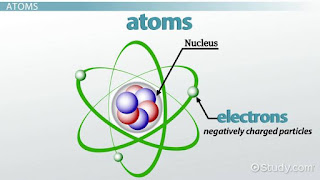[Chemisry II] Atom , Mole , Formulae And Equations - DaOptimistic World

## Monday, 3 February 2020Atoms

3.0 ATOM , MOLE , FORMULAE , EQUATIONS

Matter is made up of discrete particles, the main ones being atoms, molecules, ions.
The ancient greek were the first to use the word atom, which means indivisible, to describe the smallest particles of any substance. its actual existentce was not established until the nineteeth century when John Dalton, an English chemist, put foward a theory to describe the nature of the atom. The atom is now considered to be the basic uint of simple substances or elements.

3.1 SUMMARY (DEFINITIONS)

* ATOM is the smallest particle of an element which can take part in a chemical reaction.
* MOLECULE is the smallest particles of a substance that can normally exist alone and still retain the chemical properties of that substance.
* ION is any atom or group of atoms which possesses an electric charge.

All atoms of elements are represented by symbols, ions, molecule and radical are represented by formulae.

* ATOMICITY is the number of atoms in a single molecule of an element.
* RADICAL is a group of atom that functions as a sinle unit in chemical reactions.

3.2 RELATIVE ATOMIC & MOLECULAR MASS (RAM)&(RMM)

The Relative Atomic Mass A of an element or a compound is the number of times the average mass of one atom of that element is heavier that one-twelfth the mass of one atom of carbon-12.
>> Formuar = ( average mass of 1 atom of oxygen / 1/12 mass of 1 atom of carbon-12 )

The Relative Molecular Mass M of an element or a compound is the number of time the average mass of one molecule of it is heavier than one-twelfth the mass of one atom of carbon-12.

3.3 WORKED EXAMPLE

(A) Calculate the RMM of limestone CaCO3 When ( Ca = 40 , C = 12 O = 16 )

Solution: One molecule of CaCO3 has [1 Ca] , [1 C] and [3 O] Atoms,
therefore - The RMM of CaCO3 =
= (1 x Ca) + (1 x C) + (3 x O),
= (1 x 40) + (1 x 12) + (3 x 16),
= (40 + 12 + 48),
= 100

(B) What is the mass of 3 mole of oxygen, gas O2 ? When (O = 16)

Solution: Mass of 1 mole of O2 = (2 x 16)g = 32g
Mass of 3 mole of O2 = (3 x 16)g = 96g

(C) How many atoms are there in 6g of Carbon, When (1 mole = 6 x 10^23 , C = 12)

Solution: 12g of C = 1 mole
= 6g of C = 6/12 mole = 1/2 mole
Number of atom 1/2 in mole of c
= 6 x 10^23 / 2
= 3 x 10^23

(D) How many moles are there in 20g of CaCO3 ? When ( CaCO3 = 100)

Solution: Molar mass of CaCO3 = 100g
100g of CaCO3 = 1mole
= 20g of CaCO3,
= 20/100 X 1 mole
= 0.2 mole

(E) Calculate the percentage by mass of Nitrogen in HNO3. When (H = 1, N= 14, 0= 16)

Solution: RMM of HNO3 = 63g
63g of  HNO3 Contains 14g of Nitrogen
% by mass = 14/63 x 100
= 22.2%

3.4 THE LAW'S DEFINITIONS

* Law of conservation of mass states that matter can neither be created nor destroyed during chemical reaction but can changes from one form to another.

* Law of definite proportion states that all pure sample of a particluar chemical compound contain similar element combined in the same proportion by mass.

* Law of multiple proportions states that if two element combine to form more than one chemical compound, then the various masses of one element which combine seperately with a fixed mass of the other element are in a simple multiple ratio.

* Law of reciprocal proportion states that the masses of several elements A,B,C which combine seperately with a fixed mass of another element D, are the same as or simple multiples of the masses in which A,B,C themselves combine with one another.

3.5 VALENCY

Valency is the combining power of an element or radical. (it is usually the oxidation number assigned to the element or group of elements.)

Chemical equations are brief representation of chemical reactions using only formulae and symbols. A balanced chemical equation gives the stoichiometry of the reaction, i.e the relationship between the amounts of the reactants and products in terms of mole ratio and mass ratio. The numerical coefficients in a balanced equation give the number of moles of reactants and products, enabling easy calculations of mole and mass ratios.

POSSIBLE QUESTIONS

 What is Law of Multiple proportions ?
 What is Valency ?
 What is Atom ?
 What is ions ?
 What is molecule ?
 What is Relative Atomic Mass ?
 What is Relative Molecular Mass ?
 What is Law of reciprocal proportion ?
 What is Law of conservation of mass ?
 Matter is made of how many particles, list them ?
 What is atomicity ?
 What is law of definit proportion ?
 What is RMM of NaNO3. When (Na= 23, N= 14, O= 16) ?
 What is % by Mass of O in the NaNO3 ?
 What is % by mass of Na in the NaNO3 ?
 What is % by mass of N in the NaNO3 ?
 How many mole are there in 5g of NaNO3 ?
 Atomicity of Ozone is ?
 What is % by mass of O in Sulphur IV Oxide. When (S= 32, O= 16)